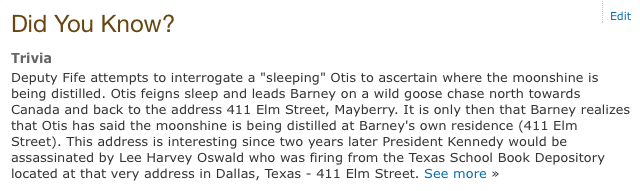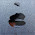## Monday, July 20, 2015

### 93 108 411 | The Andy Griffith Show & the Assassination of John F. KennedyAssassin = 411 (Jewish Gematria)

How about that, on December 25, 1961, Christmas Day, the assassination of JFK was foretold.  What a present.  Notice the episode has to do with "moonshine" and the Kennedy family came up on bootlegging, at least so we're told.
• 12/25/1961 = 1+2+2+5+1+9+6+1 = 27
• 12/25/1961 = 12+25+19+61 = 117
• 12/25/1961 = 12+25+(1+9+6+1) = 54
• 12/25/61 = 12+25+61 = 98
The episode was titled Sheriff Barney.
• Sheriff = 1+8+5+9+9+6+6 = 44/53
• Barney = 2+1+9+5+5=7 = 29
• Sheriff Barney = 73/82
• Sheriff = 19+8+5+18+9+6+6 = 71
• Barney = 2+1+18+14+5+25 = 65
• Sheriff Barney = 136
Of course it was 'The Andy Griffith Show'.
• The = 2+8+5 = 15
• Andy = 1+5+4+7 = 17
• Griffith = 7+9+9+6+6+9+2+8 = 56
• Show = 1+8+6+5 = 20/29
• The Andy Griffith Show = 108/117
Notice the episode was directed by Robert Sweeney.
• Robert = 9+6+2+5+9+2 = 33
• Sweeney = 1+5+5+5+5+5+7 = 33/42
• Robert Sweeney = 66/75
The fictional setting with the address that connects to the JFK assassination is 'Mayberry'.
• Mayberry = 4+1+7+2+5+9+9+7 = 44
• Mayberry = 13+1+25+2+5+18+18+25 = 107
Notice the '44' Gematria, or the 'kill number'.

Also, the name, 'Deputy Fife'.
• Deputy = 4+5+7+3+2+7 = 28
• Fife = 6+9+6+5 = 26
• Deputy Fife = 54 (Obama turns 54 August 4)
• Deputy = 4+5+16+21+20+25 = 91
• Fife = 6+9+6+5 = 26
• Deputy Fife = 117
• The Andy Griffith Show = 117
From Obama's birthday, August 4, 1961, to the debut of this episode, Christmas, 1961, was a span of 144-days, including the end date.

Notice the relationships between '44' and '144' below, with numbers, letters, and even the calendar.

In light of the gematria connections, 'Beast' and 'Time' both have matching gematria.  Remember, if you sum the first 144 decimal points of Pi, it totals 666.  "66, the number of the beast".

Here's another good one.

Dealey Plaza = 108
The Andy Griffith Show = 108

The name of the show also connects to propaganda and Saturn, the keeper of time.

1.From Washington to Harrison is a span of 44 years, or from the start of Washington's Presidency, 52 years..

2.Another Josh Stoss post adds that out of the 8 Presidents to die in office...4 of them were from Ohio.

8 & 4 - very much like Obama's birth date. Also Ohio = 117

To Die in Office = 296 Blood Moon = 296. From the Blood on 9/28 to the 296th day of the year Oct 23 is 25 days - my 52 alarm is going off. 10/23 is a 33 day.

3.Wow. That's just blatant.

Nice job Zach.

4.Ok the 411 elm and the moonshine connection was enough for me...but that 144 day gap from obamas bday to the episode...holy shit. The web of connections they have made is so crazy...i think i've been studying it too much, i'm almost starting to admire how intricate it all is!
5.